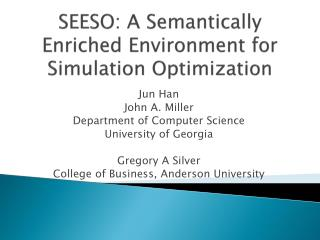# SEESO: A Semantically Enriched Environment for Simulation Optimization - PowerPoint PPT PresentationDownload PresentationSEESO: A Semantically Enriched Environment for Simulation Optimization

SEESO: A Semantically Enriched Environment for Simulation Optimization
Download Presentation## SEESO: A Semantically Enriched Environment for Simulation Optimization

- - - - - - - - - - - - - - - - - - - - - - - - - - - E N D - - - - - - - - - - - - - - - - - - - - - - - - - - -
##### Presentation Transcript

1. SEESO: A Semantically Enriched Environment for Simulation Optimization Jun Han John A. Miller Department of Computer Science University of Georgia Gregory A Silver College of Business, Anderson University

2. Outline • Introduction • Simulation Optimization (SO) • Using SO for Glycomics • Overview of Glycomics • Glycan Quantification • Metabolic Pathways • Techniques for Simulation Optimization • SESSO Framework • Two Scenarios • Conclusions

3. Gaps Among… • Conceptual model • Domain Modeling • Simulation Designing and Execution • Decision Parameter Optimization

4. Simulation Optimization • History of Simulation Optimization from 1987 • 1987: “An art, not a science” • 1998: Systematic survey and introduction • 2000: A sub-chapter in simulation textbooks • Numerous application and research on how to integrate optimization and simulation • 2011: Regular track on Simulation Optimization in WSC 2011

5. Simulation Optimization Categories • Random search methods • Random walk, Simulated Annealing • Gradient based methods • Steepest descent, Conjugate gradient, BFGS • Heuristic methods • Genetic algorithm, Particle Swarm Optimization • Meta-modeling methods • Response surface methodology • Sample path optimization • Monte Carlo Simulation

6. Using SO for Glycomics • Glycan • produced by linking saccharides and attached to proteins and lipids • Possible Applications • Cell differentiation • Disease processes • Cancer Markers • Glycomics • “an integrated systems approach to structure-function relationships of glycans” • Identification • Quantification

7. The Big Picture Omics Overview. http://jdr.sagepub.com/citmgr?gca=spjdr;90/5/561

8. Glycan Quantification. How? • Label-free methods • Isotopic labeling • Static IDAWG™ • Dynamic IDAWG™ • Mass Spectrometry • Modeling • Simulation • Optimization • Statistics Experiments Analysis

9. Quantitative Glycomics Workflow

10. Metabolic Pathway • Metabolism • Biochemical reactions • Metabolic Network GalNAc (mucin-type) core synthesis/branching http://www.ccrc.uga.edu/~moremen/glycomics/OglycanBranching/OglycanBranching/OglycanBranching.htm

11. Techniques for Simulation Optimization • SEESO: A Semantically Enriched Environment for Simulation Optimization • Bootstrapped by • JSIM: web-based simulation environment • ScalaTion: simulation environment using domain-specific language (DSL) • DeMO: Discrete-event Modeling Ontology • SoPT: Simulation oPTimizationontology

12. Overview

13. Problems and Our Solution

14. General Simulation Optimization Formula • Use Common Random Number (CRN) to reduce variance • Independent replications • Batch Means • Ranking and Selection

15. General Simulation Optimization Architecture • Simulator, Optimizer and (possible) Cost Analyzer • Loosely Coupled • Iterative approach

16. A Simple Example… def solve (x0: VectorD): VectorD = { varx = x0 // current point varxx: VectorD = null // next point vargr: VectorD = null // gradient breakable { for (k <- 1 to MAX_ITER) { // determine direction search gr = if (usePartials) gradientD (df, x) // use functions for partials else gradient (fg, x) xx = lineSearch (x, gr) if (abs (fg(xx) - fg(x)) < EPSILON) break x = xx }} // for x } // solve Objective Function Steepest Descent, etc.

17. Simulation oPTimization (SoPT) Ontology • Establish connection between numerous real world problems and optimization algorithms • Top level classes: • Optimization Component • Optimization Problem • Optimization Method

18. Optimization Component in SoPT

19. Optimization Problem in SoPT

20. Optimization Method in SoPT

21. DeMO + SoPT + DSL + Rule

22. Rule-based Algorithm selection • A set of Rules • Rule inferencing (Rete algorithm)

23. Optimization algorithm configuration and execution • Automatic Algorithm Configuration • Algorithm execution using DSL

24. Scenario 1: Urgent Care Facility • Model definition using DeMO • Code generation using ScalaTion DSL • Optimization algorithm selection using SoPT • Optimization execution using DSL

25. Urgent Care Facility (cont.)

26. Scenario 2: Metabolic Pathway • Substrate (E), Product (P), Enzyme (E) • Decision Parameters • Rate constants • Temperatures, Enzyme concentration, gene expression level, etc. 2 1 3 4 5

27. Metabolic Pathway Models:Petri Net and Systems of Differential Equations

28. Conclusions • Quantitative glycomics needs simulation optimization • Integration of ontology and DSL can facilitate modeling, simulation and application of simulation optimization for domain modelers

29. Questions?